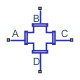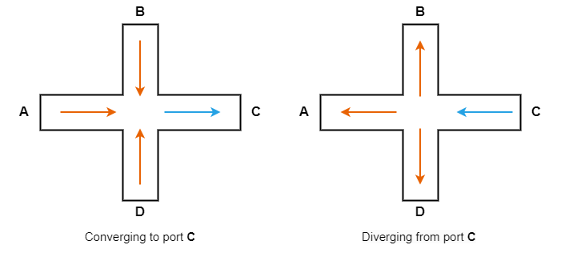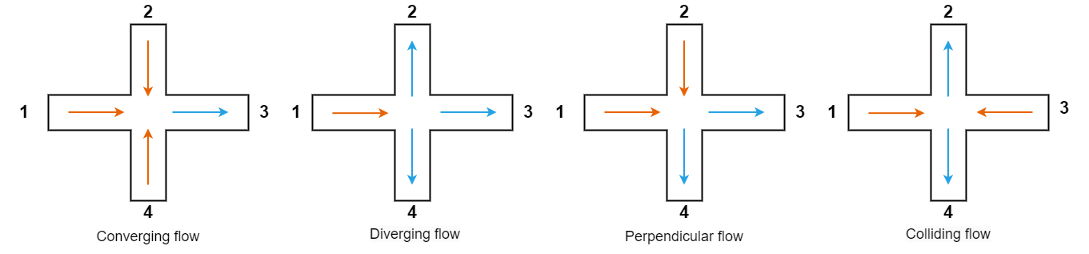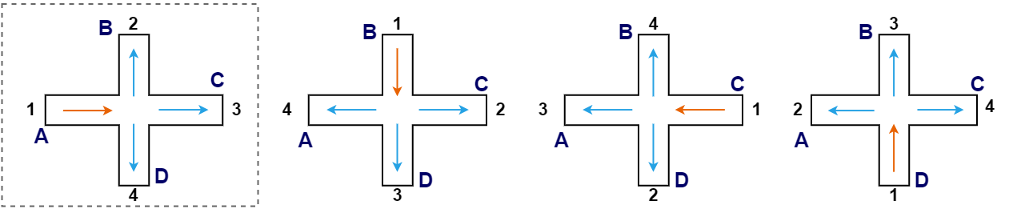# Cross-Junction (IL)

Cross-junction in an isothermal liquid network

Since R2022b

•Libraries:
Simscape / Fluids / Isothermal Liquid / Pipes & Fittings

## Description

The Cross-Junction (IL) block represents a four-way junction in an isothermal liquid network. The block abstracts the fluid interactions depending on the direction of flow at each port, where ports A and C are the main line and ports B and D are the branch line.

### Mass and Momentum Balance

The block conserves mass such that

`${\stackrel{˙}{m}}_{A}+{\stackrel{˙}{m}}_{B}+{\stackrel{˙}{m}}_{C}+{\stackrel{˙}{m}}_{D}=0,$`

where i is the mass flow rate for a given port, i.

The block determines which coefficient and vector element to use, then computes the pressure difference to conserve momentum such that

`${p}_{i}-{p}_{1}={I}_{i}+\frac{{k}_{i}}{2}{\stackrel{˙}{m}}_{i}\sqrt{{\stackrel{˙}{m}}_{i}^{2}+{\stackrel{˙}{m}}_{threshold}^{2}}}{\overline{\rho }{A}_{i}^{2}},$`

where:

• $\overline{\rho }$ is the average fluid density.

• pi is the pressure for a given port, i.

• p1 is the pressure at node 1.

• ki is the flow coefficient that the block assigns to a given port, i. The Loss coefficient model parameter specifies the block method used to calculate ki.

• Ii is the fluid inertia for a given port, i.

The block calculates the inertia for each port as

`$\begin{array}{l}{I}_{A}={\stackrel{¨}{m}}_{A}\frac{\sqrt{\pi {A}_{side}}}{{A}_{main}}\\ {I}_{B}={\stackrel{¨}{m}}_{B}\frac{\sqrt{\pi {A}_{main}}}{{A}_{side}}\\ {I}_{C}={\stackrel{¨}{m}}_{C}\frac{\sqrt{\pi {A}_{side}}}{{A}_{main}}\\ {I}_{D}={\stackrel{¨}{m}}_{D}\frac{\sqrt{\pi {A}_{main}}}{{A}_{side}}\end{array}$`

where Amain is the value of the Main line area (A,C) parameter and Aside is the value of the Branch line area (B,D) parameter.

### Idel'chik Correlation Coefficient Model

When you set the Loss coefficient model parameter to `Idel'chik correlation`, the block calculates the pipe loss coefficients according to . The Idel'chik model only supports flow converging to port C or flow diverging from port C. To achieve expected results, orient the block appropriately to the expected flow configuration of the model fluid network.

The block treats the junction as symmetrical about both the horizontal and vertical planes such that AA = AC is the value of the Main line area (A,C) parameter, and AB = AD is the value of the Branch line area (B,D) parameter, where AA,B,C,D is the area of the corresponding port.In the case of a prohibited flow geometry, you can control the block behavior by using the Report when flow configuration is invalid parameter. If the Report when flow configuration is invalid parameter is `None` or `Warning`, the model continues to run in the prohibited flow configuration, but the results may not be correct.

Converging Flow to Port C

When the flow is converging to port C, the loss coefficients for ports A and C are

`$\begin{array}{l}{k}_{A}=\left(1+{\left(\frac{{\stackrel{˙}{m}}_{A}}{{\stackrel{˙}{m}}_{C}}\right)}^{2}-{\left(\frac{{\stackrel{˙}{m}}_{A}}{{\stackrel{˙}{m}}_{C}}\right)}^{2}\frac{1+\frac{{\stackrel{˙}{m}}_{A}}{{\stackrel{˙}{m}}_{C}}}{{\left(0.75+0.25\frac{{\stackrel{˙}{m}}_{A}}{{\stackrel{˙}{m}}_{C}}\right)}^{2}}\right){\left(\frac{{\stackrel{˙}{m}}_{A}}{{\stackrel{˙}{m}}_{C}}\frac{{A}_{C}}{{A}_{A}}\right)}^{-2}\\ {k}_{C}=0\end{array}$`

The converging flow loss coefficients for ports B and D are

`$\begin{array}{l}{k}_{B}=\left(1+{\left(\frac{{\stackrel{˙}{m}}_{B}}{{\stackrel{˙}{m}}_{C}}\frac{{A}_{C}}{{A}_{B}}\right)}^{2}-8{\left(\frac{{\stackrel{˙}{m}}_{B}}{{\stackrel{˙}{m}}_{C}}\right)}^{2}\frac{{\left[\frac{{\stackrel{˙}{m}}_{C}}{{\stackrel{˙}{m}}_{B}}-\left(1+\frac{{\stackrel{˙}{m}}_{D}}{{\stackrel{˙}{m}}_{B}}\right)\right]}^{2}}{4-\left(1+\frac{{\stackrel{˙}{m}}_{D}}{{\stackrel{˙}{m}}_{B}}\right)\frac{{\stackrel{˙}{m}}_{B}}{{\stackrel{˙}{m}}_{C}}}\right){\left(\frac{{\stackrel{˙}{m}}_{B}}{{\stackrel{˙}{m}}_{C}}\frac{{A}_{C}}{{A}_{B}}\right)}^{-2}\\ {k}_{D}=\left(1+{\left(\frac{{\stackrel{˙}{m}}_{D}}{{\stackrel{˙}{m}}_{C}}\frac{{A}_{C}}{{A}_{D}}\right)}^{2}-8{\left(\frac{{\stackrel{˙}{m}}_{D}}{{\stackrel{˙}{m}}_{C}}\right)}^{2}\frac{{\left[\frac{{\stackrel{˙}{m}}_{C}}{{\stackrel{˙}{m}}_{D}}-\left(1+\frac{{\stackrel{˙}{m}}_{B}}{{\stackrel{˙}{m}}_{D}}\right)\right]}^{2}}{4-\left(1+\frac{{\stackrel{˙}{m}}_{B}}{{\stackrel{˙}{m}}_{D}}\right)\frac{{\stackrel{˙}{m}}_{D}}{{\stackrel{˙}{m}}_{C}}}\right){\left(\frac{{\stackrel{˙}{m}}_{D}}{{\stackrel{˙}{m}}_{C}}\frac{{A}_{C}}{{A}_{D}}\right)}^{-2}\end{array}$`

Diverging Flow From Port C

When the flow diverges from port C, the loss coefficients for ports A and C are

`$\begin{array}{l}{k}_{A}=0.4{\left(\frac{{\stackrel{˙}{m}}_{C}}{{\stackrel{˙}{m}}_{A}}-1\right)}^{2}\\ {k}_{C}=0\end{array}$`

The diverging flow loss coefficients for ports B and D are

`$\begin{array}{l}{k}_{B}=A\text{'}{\left(\frac{{\stackrel{˙}{m}}_{C}}{{\stackrel{˙}{m}}_{B}}\frac{{A}_{B}}{{A}_{C}}+1\right)}^{2}\\ {k}_{D}=A\text{'}{\left(\frac{{\stackrel{˙}{m}}_{C}}{{\stackrel{˙}{m}}_{D}}\frac{{A}_{D}}{{A}_{C}}+1\right)}^{2}\end{array}$`

where A'=1 when and A'≈0.9 when . The block smooths the transition between A' = 1 and A' = 0.9 using a hyperbolic tangent function.

According to , these loss coefficients are only applicable for diverging flow when the ratio of the side branch diameter to the main branch diameter is less than or equal to `2/3`. However, the expected error for ratios above `2/3` is generally low enough that the block continues to use the above loss coefficients.

### Custom Cross-Junction

When you set the Loss coefficient model parameter to `Custom`, the block uses the user-defined loss parameters to describe the pipe loss coefficient at each port.

For a custom cross-junction, the block allows four configurations diverging flow, converging flow, perpendicular flow, and colliding flow:

• Diverging flow — Flow enters at node 1 and exits at nodes 2, 3, and 4.

• Converging flow — Flow enters at nodes 1, 2, and 4 and exits at node 3.

• Perpendicular flow — Flow enters at nodes 1 and 2 and exits at nodes 3 and 4.

• Colliding flow — Flow enters at nodes 1 and 3 and exits at nodes 2 and 4.

This figure demonstrates these configurations.The block treats the junction as symmetrical about both the horizontal and vertical planes such that A1 = A3 and A2 = A4, where A1,2,3,4 is the area of the corresponding port in the figure. Using this symmetry, the block assumes the loss coefficients from 1 to 2 and from 1 to 4 are identical during diverging, converging, and colliding flow.

During simulation, the block continually checks the direction of flow at each port and compares the result to the four possible flow configurations. When the block determines the flow configuration, it adjusts the node that each port corresponds to. For example, when the block experiences diverging flow where the fluid enters at port A and exits at ports B, C, and D, it assigns node 1 to port A as in the first panel of the figure. The other panels show the assigned nodes for diverging flow entering at ports other than A.For the custom cross-junction, all four flow configurations can occur in the same block during a simulation. You only need to supply parameters for each condition that the block experiences. If you use two-element vectors to specify the flow coefficients, the block uses the first or second element depending on whether node 1 aligns with the main line or the branch line. The first element corresponds to node 1 at port A or C, and the second element corresponds to node 1 at port B or D.

This table describes the conditions and coefficients for each operational mode used to determine the loss coefficients.

Flow ScenarioABCDkAkBkCkD
Diverging from node A>thresh<-ṁthresh<-ṁthresh<-ṁthresh0kdiv,turning,mainkdiv,straight,mainkdiv,turning,main
Diverging from node B<-ṁthresh>thresh<-ṁthresh<-ṁthreshkdiv,turning,side0kdiv,turning,sidekdiv,straight,side
Diverging from node C<-ṁthresh<-ṁthresh>thresh<-ṁthreshkdiv,straight,mainkdiv,turning,main0kdiv,turning,main
Diverging from node D<-ṁthresh<-ṁthresh<-ṁthresh>threshkdiv,turning,sidekdiv,straight,sidekdiv,turning,side0
Converging to node A<-ṁthresh>thresh>thresh>thresh0kconv,turning,mainkconv,straight,mainkconv,turning,main
Converging to node B>thresh<-ṁthresh>thresh>threshkconv,turing,side0kconv,turing,sidekconv,straight,side
Converging to node C>thresh>thresh<-ṁthresh>threshkconv,straight,mainkconv,turning,main0kconv,turning,main
Converging to node D>thresh>thresh>thresh<-ṁthreshkconv,turing,sidekconv,straight,sidekconv,turing,side0
Perpendicular with main entry A>thresh>thresh<-ṁthresh<-ṁthresh0kperp,turning in,mainkperp,straight,mainkperp,turning out,main
Perpendicular with main entry B<-ṁthresh>thresh>thresh<-ṁthreshkperp,turning out,side0kperp,turning in,sidekperp,turning straight,side
Perpendicular with main entry C<-ṁthresh<-ṁthresh>thresh>threshkperp,straight,mainkperp,turning out,main0kperp,turning in,main
Perpendicular with main entry D>thresh<-ṁthresh<-ṁthresh>threshkperp,turning in,sidekperp,turning straight,sidekperp,turning out,side0
Colliding from main to branch >thresh<-ṁthresh>thresh<-ṁthresh0kcoll,turning,mainkcoll,straight,mainkcoll,turning,main
Colliding from branch to main<-ṁthresh>thresh<-ṁthresh>threshkcoll,turning,side0kcoll,turning,sidekcoll,straight,side
Stagnant1 or last valid1 or last valid1 or last valid1 or last valid

The flow is stagnant when the mass flow rate conditions do not match any defined flow scenario. The block uses these parameters to calculate the flow coefficients:

• kdiv,straight,main and kdiv,straight,side are the first and second elements of the Diverging flow straight loss coefficient parameter, respectively.

• kdiv,turning,main and kdiv,turning,side are the first and second elements of the Diverging flow turning loss coefficient parameter, respectively.

• kconv,straight,main and kconv,straight,side are the first and second elements of the Converging flow straight loss coefficient parameter, respectively.

• kconv,turning,main and kconv,turning,side are the first and second elements of the Converging flow turning loss coefficient parameter, respectively.

• kperp,straight,main and kperp,straight,side are the first and second elements of the Perpendicular flow straight loss coefficient parameter, respectively.

• kperp,turning in,main and kperp,turning in,side are the first and second elements of the Perpendicular flow straight loss coefficient parameter, respectively.

• kperp,turning out,main and kperp,turning out,side are the first and second elements of the Perpendicular flow straight loss coefficient parameter, respectively.

• kcoll,straight,main and kcoll,straight,side are the first and second elements of the Colliding flow straight loss coefficient parameter, respectively.

• kcoll,turning,main and kcoll,turning,side are the first and second elements of the Colliding flow turning loss coefficient parameter, respectively.

### Variables

To set the priority and initial target values for the block variables prior to simulation, use the Initial Targets section in the block dialog box or Property Inspector. For more information, see Set Priority and Initial Target for Block Variables.

Nominal values provide a way to specify the expected magnitude of a variable in a model. Using system scaling based on nominal values increases the simulation robustness. Nominal values can come from different sources, one of which is the Nominal Values section in the block dialog box or Property Inspector. For more information, see Modify Nominal Values for a Block Variable.

## Ports

### Conserving

expand all

Isothermal liquid conserving port associated with the liquid port..

Isothermal liquid conserving port associated with the liquid port..

Isothermal liquid conserving port associated with the liquid port..

Isothermal liquid conserving port associated with the liquid port..

## Parameters

expand all

### Junction Properties

Cross-sectional area of the main flow path from port A to port C.

Cross-sectional area of the branch flow path from port B to port D.

Upper Reynolds number limit for laminar flow through the junction.

Junction loss coefficient model. Set this parameter to `Custom` to specify individual diverging and converging loss coefficients for each flow path segment.

Simulation warning mode for operating conditions outside the valid flow configurations. If you select `Warning`, the block generates a warning for conditions outside the valid flow configurations. The warning does not cause simulation to stop. If you select `Error`, the simulation will stop for conditions outside the valid flow configurations.

#### Dependencies

To enable this parameter, set Loss coefficient model to ```Idel'chik correlation```.

Whether to specify loss coefficients for diverging flow.

#### Dependencies

To enable this parameter, set Loss coefficient model to `Custom`.

Whether to specify loss coefficients for converging flow.

#### Dependencies

To enable this parameter, set Loss coefficient model to `Custom`.

Whether to specify loss coefficients for perpendicular flow.

#### Dependencies

To enable this parameter, set Loss coefficient model to `Custom`.

Whether to specify loss coefficients for colliding flow.

#### Dependencies

To enable this parameter, set Loss coefficient model to `Custom`.

### Diverging Flow Coefficients

Loss coefficient for the straight portion of the diverging flow. When you specify this parameter as a vector, the first element represents the loss coefficient when ports A or C align to node 1. The second element represents when ports B or D align to node 1. The block behaves the same for all orientations when you use a scalar.

#### Dependencies

To enable this parameter, set Loss coefficient model to `Custom` and select Diverging flow loss coefficients.

Loss coefficient for the turning portions of the diverging flow. When you specify this parameter as a vector, the first element represents the loss coefficient when ports A or C align to node 1. The second element represents when ports B or D align to node 1. The block behaves the same for all orientations when you use a scalar.

#### Dependencies

To enable this parameter, set Loss coefficient model to `Custom` and select Diverging flow loss coefficients.

### Converging Flow Coefficients

Loss coefficient for the straight portion of the converging flow. When you specify this parameter as a vector, the first element represents the loss coefficient when ports A or C align to node 1. The second element represents when ports B or D align to node 1. The block behaves the same for all orientations when you use a scalar.

#### Dependencies

To enable this parameter, set Loss coefficient model to `Custom` and select Converging flow loss coefficients.

Loss coefficient for the turning portions of the converging flow. When you specify this parameter as a vector, the first element represents the loss coefficient when ports A or C align to node 1. The second element represents when ports B or D align to node 1. The block behaves the same for all orientations when you use a scalar.

#### Dependencies

To enable this parameter, set Loss coefficient model to `Custom` and select Converging flow loss coefficients.

### Perpendicular Flow Coefficients

Loss coefficient for the straight portion of the perpendicular flow. When you specify this parameter as a vector, the first element represents the loss coefficient when ports A or C align to node 1. The second element represents when ports B or D align to node 1. The block behaves the same for all orientations when you use a scalar.

#### Dependencies

To enable this parameter, set Loss coefficient model to `Custom` and select Perpendicular flow loss coefficients.

Loss coefficient for the flow turning to inflow in the perpendicular flow. When you specify this parameter as a vector, the first element represents the loss coefficient when ports A or C align to node 1. The second element represents when ports B or D align to node 1. The block behaves the same for all orientations when you use a scalar.

#### Dependencies

To enable this parameter, set Loss coefficient model to `Custom` and select Perpendicular flow loss coefficients.

Loss coefficient for the flow turning to outflow in the perpendicular flow. When you specify this parameter as a vector, the first element represents the loss coefficient when ports A or C align to node 1. The second element represents when ports B or D align to node 1. The block behaves the same for all orientations when you use a scalar.

#### Dependencies

To enable this parameter, set Loss coefficient model to `Custom` and select Perpendicular flow loss coefficients.

### Colliding Flow Coefficients

Loss coefficient for the straight portion of the colliding flow. When you specify this parameter as a vector, the first element represents the loss coefficient when ports A or C align to node 1. The second element represents when ports B or D align to node 1. The block behaves the same for all orientations when you use a scalar.

#### Dependencies

To enable this parameter, set Loss coefficient model to `Custom` and select Colliding flow loss coefficients.

Loss coefficient for the turning portion of the colliding flow. When you specify this parameter as a vector, the first element represents the loss coefficient when ports A or C align to node 1. The second element represents when ports B or D align to node 1. The block behaves the same for all orientations when you use a scalar.

#### Dependencies

To enable this parameter, set Loss coefficient model to `Custom` and select Colliding flow loss coefficients.

 Idel’chik, I. E. Handbook of hydraulic resistance: Coefficients of local resistance and of friction. Jerusalem: Israel Program for Scientific Translations, 1966.

 Idel’chik, I. E. Handbook of hydraulic resistance: Coefficients of local resistance and of friction. Jerusalem: Israel Program for Scientific Translations, 1986.